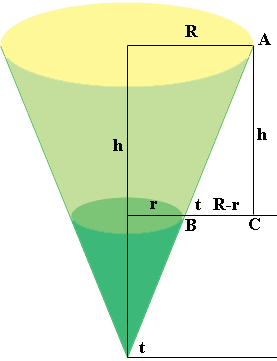SEARCH HOMEMath Central Quandaries & QueriesQuestion from CV: If I know the height, taper, and volume of a frustum cone, what are its Radii? Calculating frustum cone volume is straight forward. v=Pi/3*h*(R*R+r*r+R*r) where; v=volume; h=frustum height; R=major radius; r=minor radius; Pi=3.1415926; t=taper, ('slant angle' where t=0 is a disk) Here is the tricky part. Knowing 'h', 't', & 'v'; Calculate one or both of 'R' & 'r'. Specifically, what is the formula for 'R=' or 'r='? This is MUCH harder than you may think. Thanks, CVHi,

I drew a diagram of the frustum of a cone and added some lines and labels.From the triangle $ABC$ in the diagram you can see that $\tan t = \frac{h}{R-r}.$ Solving for $R$ gives

$R = r + h \tan t.$

Substitute into the volume expression

$v= \frac{\pi}{3} \times h \times (R \times R + r \times r+ R \times r).$

This will give a quadratic in $r$. Solve for $r.$

PennyMath Central is supported by the University of Regina and The Pacific Institute for the Mathematical Sciences.